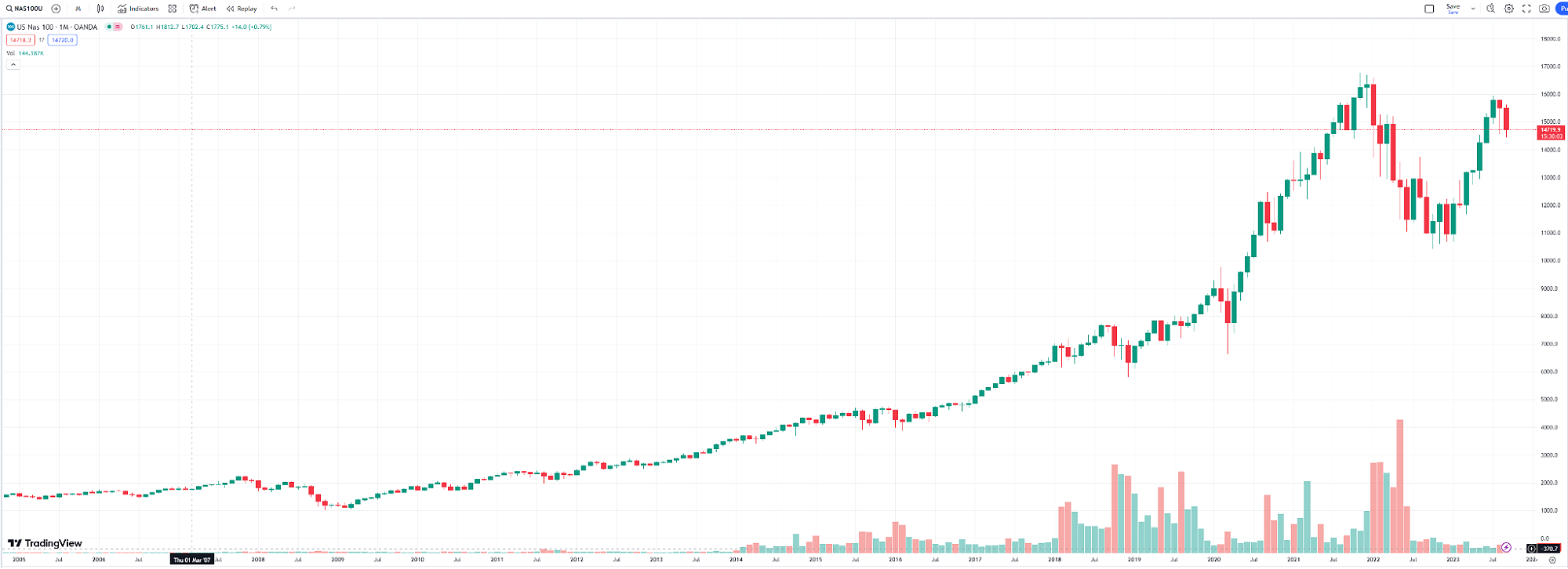Choose another country or region to see content specific to your location# What is a stock market index and how are they calculated?OANDA
Team

### What is a Stock Market Index?

A 'stock market index' or 'stock index' is a way of measuring the performance of a group of stocks, usually categorized by country, company size, or industry. As an example, the Nasdaq-100 is an index made up of the 100 largest non-financial stocks in the US, including the likes of Meta, Tesla, and Apple as 'constituent stocks'.

Investors can analyze the value of a stock index over time to better understand the relative performance of a given industry, country, or both.

Generally speaking, a stock index increasing in price means the market, as a whole, is gaining value, and market participants are likely to be more optimistic about future growth. In contrast, if the price of a stock index were to decrease, the opposite could be said.

The following chart shows the performance of the Nasdaq-100 over the past five years of trading:### How can the value of a Stock Index be Calculated?

The value of a stock index can be calculated in a number of ways, but one method is to find the average value of the stocks it includes.

For example, supposing the 'ABC index' includes shares of both Amazon (\$100 per share), Microsoft (\$200 per share), and Apple (\$500 per share), the following calculation would apply:

(Amazon share value + Microsoft share value + Apple share value)

/3 (divided by the number of stocks in the index)

=

ABC index value

(100 + 200 + 500)

/3

=

266.66

ABC index value: \$266.66

Naturally, if the stock prices of these three companies were to fluctuate, so would the value of the 'ABC index'.

That said, this means of calculating index value is not perfect. Owing to the functionality of averages, the lower the stock price, the less impact it would have on the overall value of the corresponding index.

For example, in the above scenario, if Amazon's stock price were to rise by 10% in value while the other two stocks remained unchanged:

Amazon share value + 10% = \$110

Microsoft share value = \$200

Apple share value = \$500

The value of the 'ABC index' would be as follows:

(110 + 200 + 500)

/3

= 270

ABC index value: \$270.00

But if the largest of the three stocks, Apple, were to increase by 10% in value instead while Microsoft and Amazon remain unchanged:

Amazon share value = \$100

Microsoft share value = \$200

Apple share value + 10% = \$550

The outcome would be much different:

(100 + 200 + 550)

/3

= 283.33

ABC index value: \$283.33

As stock price alone is not a perfect indicator of company valuation, this method of calculation can be flawed.

Many stock indices average the value of companies by weighting their market capitalization instead. By this method, as long as each company has a similar market value, individual stock prices can vary considerably but will have the same impact on the stock index value as a whole.